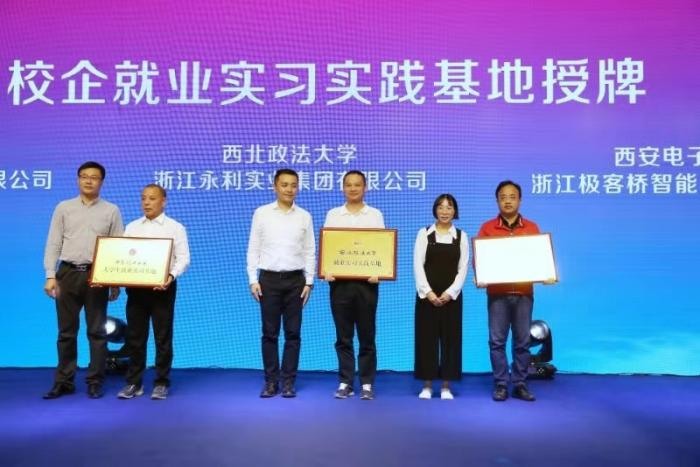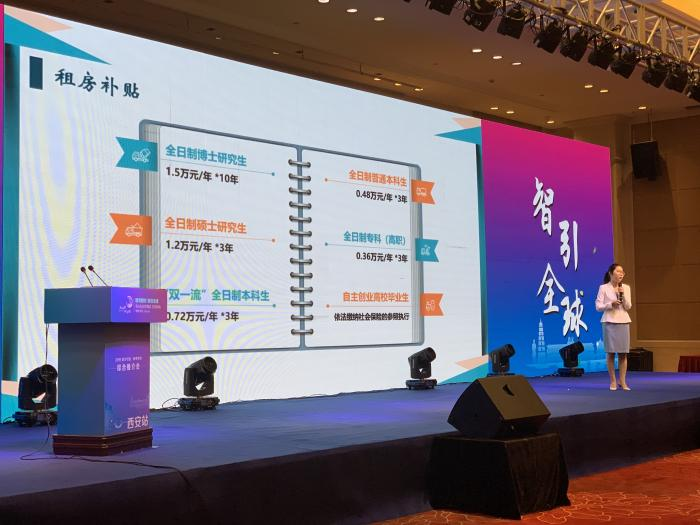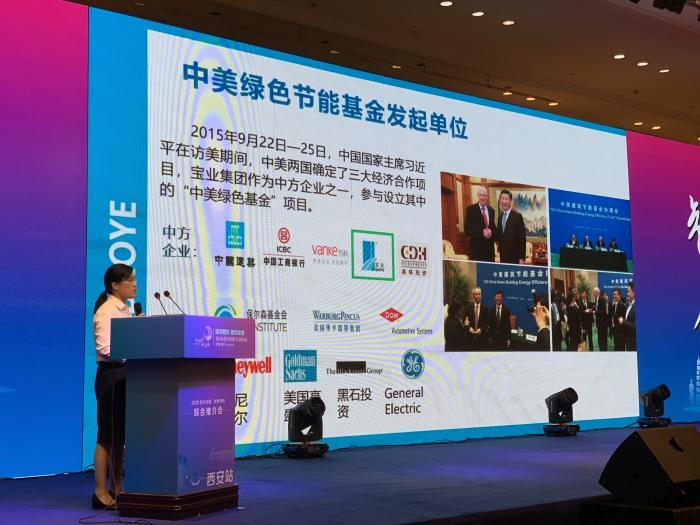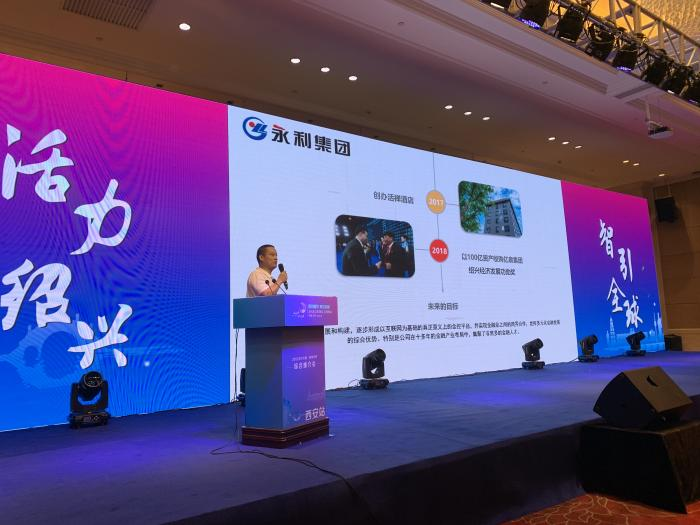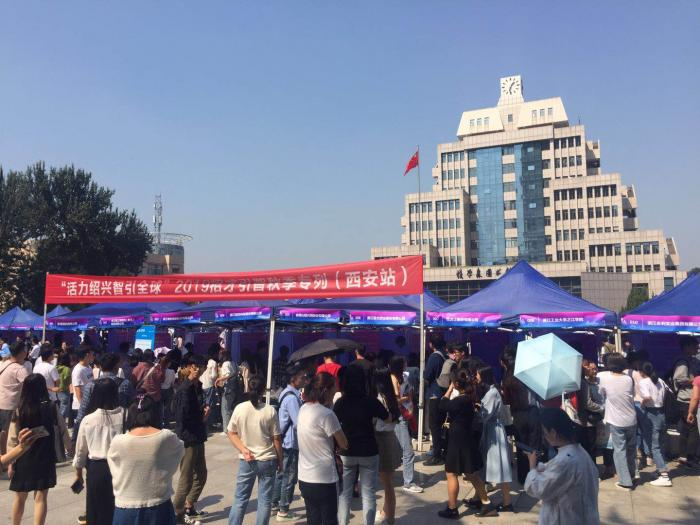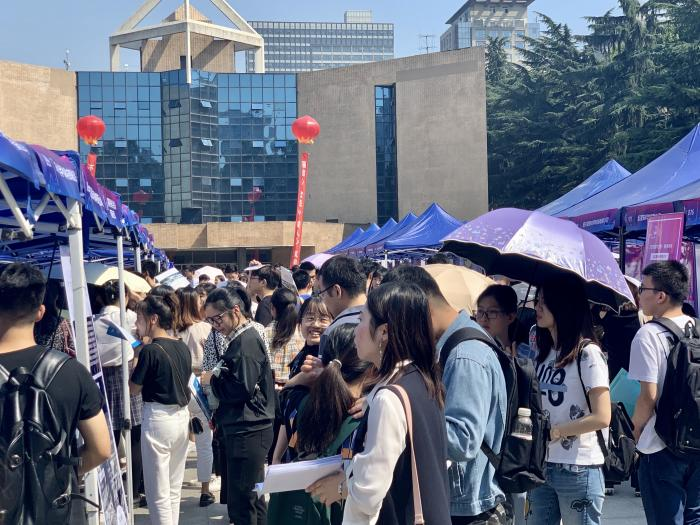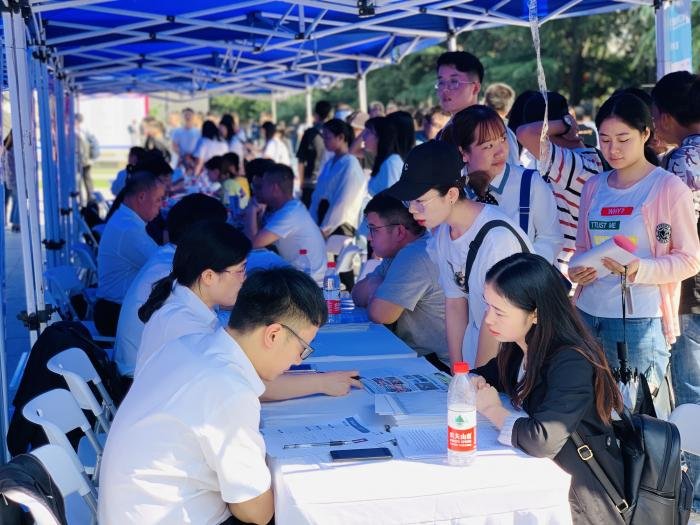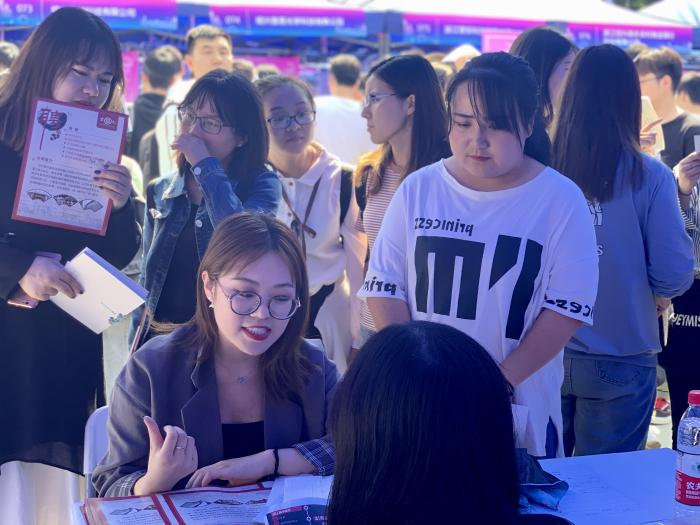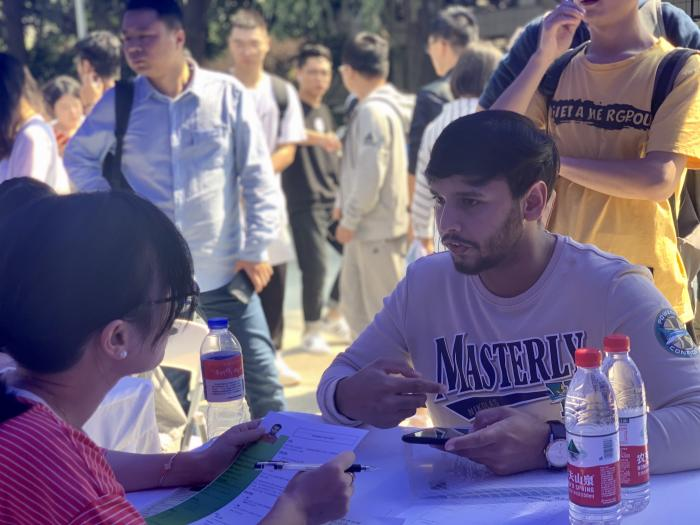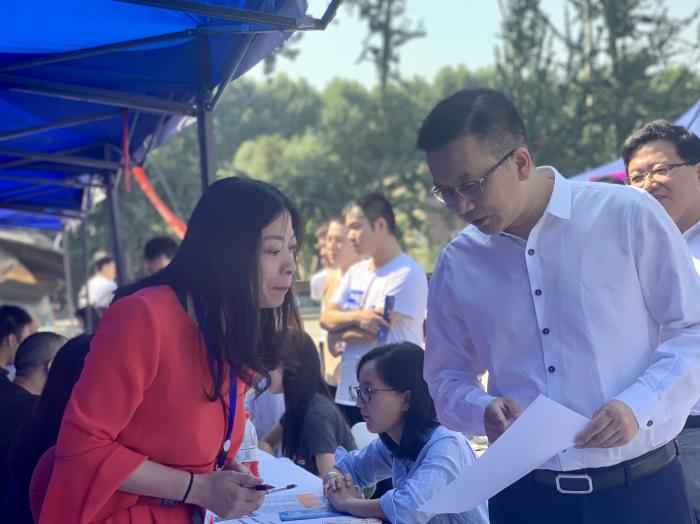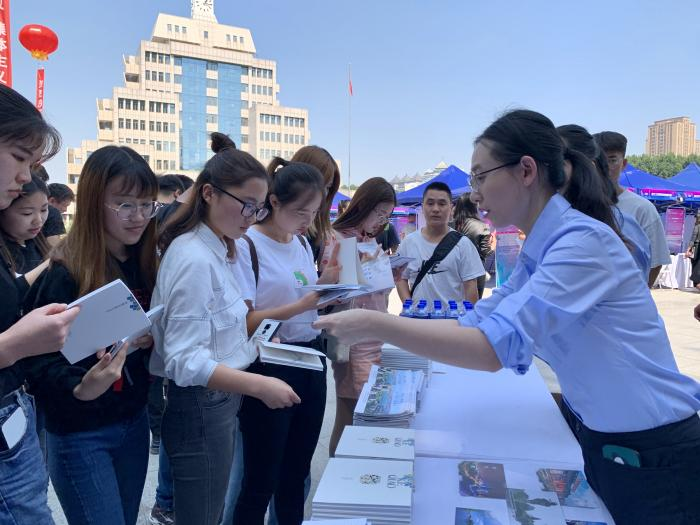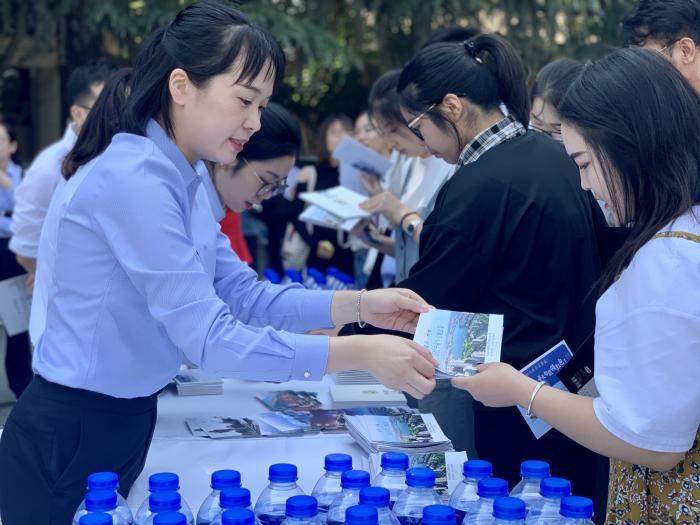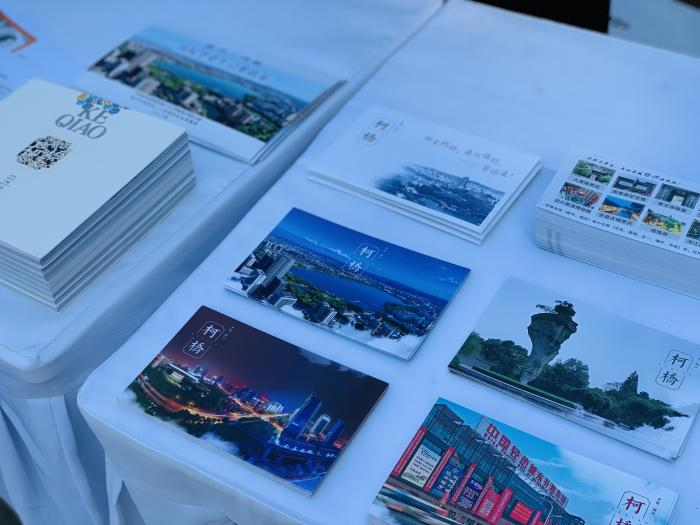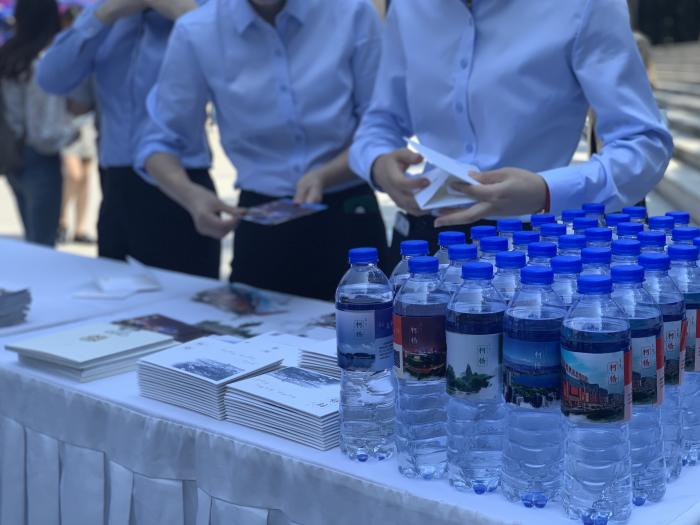#### 请选择工作岗位

• 经营管理类
•     总裁/总经理/CEO
•     技术总监/项目经理/CTO
•     营销总监
•     财务总监/CFO
•     人力资源总监
•     行政总监
•     经理助理
•     企管部经理/管理顾问
•     企业管理人员
•     信息主管CIO
•     投资管理
•     厂长/副厂长
• 计算机/IT业人员
•     系统分析员
•     软件（测试）工程师
•     硬件（测试）工程师
•     网络工程
•     网站信息编辑
•     网站策划
•     网页设计/电脑美工
•     多媒体设计与开发
•     计算机辅助设计与绘图
•     数据库开发与管理
•     系统集成/支持
•     系统安全管理
•     Internet/WEB/电子商务
• 电子/通讯类专业人员
•     电子工程师
•     电子元器件工程师
•     电子/电器维修
•     无线电技术
•     电路设计/电子测试/半导体技术
•     通讯工程师
•     自动控制
•     单片机/DLC/DSP/底层软件开发
•     仪器/仪表/计量
•     智能大厦/综合布线/弱电
•     电气
•     电力
• 销售/业务类
•     销售经理/主任
•     销售主管/代表
•     销售助理
•     商务经理/主管
•     商务代表
•     涉外业务
•     促销员
• 市场营销/公关
•     市场/营销经理
•     市场助理/专员
•     产品/品牌企划
•     市场通路企划
•     价格企划
•     广告企划
•     新闻媒介策划
•     市场推广/拓展/合作
•     市场分析/调研
•     公关经理/主管
•     公关专员
•     广告创意/设计/策划
• 客户服务类
•     客户服务经理
•     客户培训
•     客户关系管理
•     售前/售后支持
•     客户数据库维护
•     客户分析
•     客户渠道
•     投诉处理
•     投诉监控
•     热线人员
• 行政/人力资源管理类
•     行政经理
•     行政主管/助理
•     办公室主任
•     行政秘书/行政专员
•     人力资源经理
•     招聘经理/主管/专员
•     培训经理/主管/专员
•     薪酬福利经理/主管/专员
•     绩效考核经理/主管/专员
•     人事主管/助理/专员
• 文职类
•     图书情报/资料/文档管理
•     资料/文档的撰写/编辑
•     文秘/高级文员
•     文员/电脑录入/校对
•     前台/接待/礼仪
•     热线咨询
• 翻译类
•     英语
•     日语
•     法语
•     德语
•     朝鲜语
•     西班牙语
•     俄语
• 财务/审计/统计
•     财务经理/副经理/主任
•     会计师
•     注册会计师
•     会计主管
•     会计
•     财务会计
•     管理会计
•     出纳
•     审计经理/主管/专员
•     统计
• 设计类
•     美术/图形设计
•     工业/产品设计
•     工艺品设计
•     室内外装修/装潢设计
•     纺织服装设计
•     家具/珠宝设计
•     形象设计
•     电脑绘图
• 工业/工厂类
•     生产管理
•     工程管理
•     品质管理
•     物料管理
•     物流管理
•     设备管理
•     采购管理
•     仓库管理
•     计划/调度员
•     化验/检验员
•     跟单/单证员
•     PMC/SMT技术员
• 金融/经济专业人员
•     金融/投资
•     信贷/证券
•     期货经济
•     外汇
•     资产评估
•     税务经理/主管/专员
•     保险业务/经纪人
•     预结算人员
•     稽核员
•     报关员
•     船务
• 法律专业人员
•     律师
•     法律顾问
•     法务助理
• 机械专业人员
•     机械工程师
•     模具工程师
•     机电工程师
•     机械设计
•     机械制图
• 房地产/土建类专业人员
•     房地产开发/策划
•     房地产评估/交易
•     建筑/结构工程师
•     工程监理师
•     工程预决算
•     给排水/水电工程师
•     水电管理
•     建筑施工管理
•     制冷暖通
•     物业管理
•     工民建
•     路桥工程
•     管道
•     绘图/建筑制图
•     基础地下工程/岩土工程
•     港口与航道工程
• 咨询/顾问
•     咨询经理
•     咨询员
•     专业顾问
•     信息中介
•     培训师
• 医疗/护理/保健类
•     中/西医师
•     医药技术员
•     护士/护理人员
•     医/药学检验员
•     兽医/宠物医生
•     心理医生
•     药剂师
•     麻醉师
•     卫生防疫
•     妇幼保健
•     针灸推拿
•     化妆/美容师
•     化工制药
• 技工类
•     钳工/机修工/钣金工
•     电焊工/铆焊工
•     车工/磨工/铣工/冲床/锣床/车床
•     模具工
•     水工/木工/油漆工
•     电工
•     叉车工
•     空调工/电梯工/锅炉工
•     裁剪车缝熨烫
•     普工
• 文教体
•     新闻采编/记者/摄影
•     编辑
•     出版
•     高等教育
•     中等教育
•     小学/幼儿教育
•     职业教育/家教
•     体育类
•     影视策划/制作
•     表演人员
•     节目主持/DJ/播音
•     音效师
• 服务业专业人员
•     旅游/导游
•     宾馆酒店
•     娱乐餐饮
•     寻呼/声讯
•     收银员/营业员/服务员
•     理货员
•     司机
•     保安
• 纺织印染
•     家用纺织品
•     面料
•     纺织机械及器材
•     纤维原料
•     染料助剂
•     纱线
•     服装服饰
•     皮革及皮毛
•     无纺布
•     辅料
•     印染及后整理服务
•     其他
•     设计师
•     设计总监
•     产品总监
•     跟单员
•     制版师
•     样板师
•     工艺师
•     市场总监
•     销售主管
•     销售助理
•     生产厂长
•     车间主任
•     生产管理
•     采购主管
•     高级技师
•     外贸人员
•     印染工程师
•     行政人员
•     人力资源
•     财务/统计
•     翻译
•     单证员
•     技工
• 实习生

• 浙江
• 浙江/绍兴市
• 浙江/杭州市
• 浙江/宁波市
• 浙江/金华市
• 浙江/台州市
• 浙江/衢州市
• 浙江/温州市
• 浙江/嘉兴市
• 浙江/湖州市
• 浙江/舟山市
• 浙江/丽水市
• 北京
• 天津
• 河北
• 山西
• 内蒙古
• 辽宁
• 吉林
• 黑龙江
• 上海
• 江苏
• 安徽
• 福建
• 江西
• 山东
• 河南
• 湖北
• 湖南
• 广东
• 广西
• 海南
• 四川
• 贵州
• 云南
• 西藏
• 陕西
• 甘肃
• 青海
• 宁夏
• 新疆
• 其他
• 重庆
• 香港特别行政区
• 澳门特别行政区
• 台湾省 台湾省

#### 请选择发布日期

• 3天内
• 半月内
• 一个月内
• 一周内
• 三个月内
• 半年内

 ZJ1078145 大专 应用电子技术 ZJ1078132 本科 机械工程 ZJ1078097 初中 上班工作 ZJ1078095 模具设计与制造 ZJ1078092 硕士 道路工程 ZJ1078080 大专 空中乘务 ZJ1078046 本科 电气工程及其自动化 ZJ1076914 大专 会计

9月25日上午，2019招才引智·秋季专列（西安站)活动隆重启幕，在西安建国饭店闪亮登场！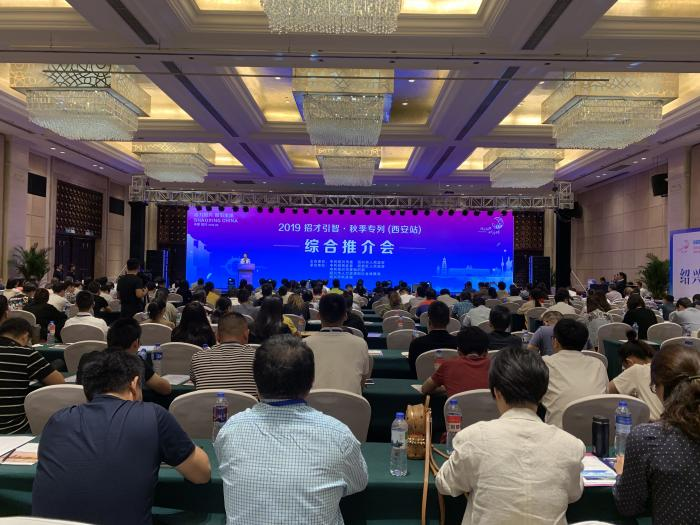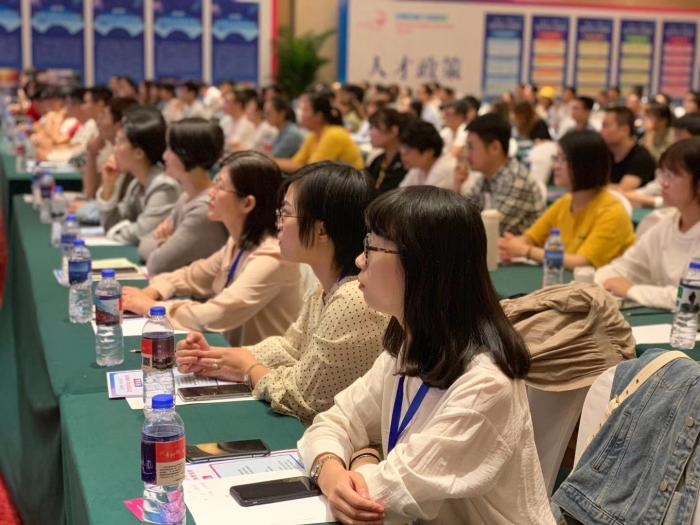1.引才联络点授牌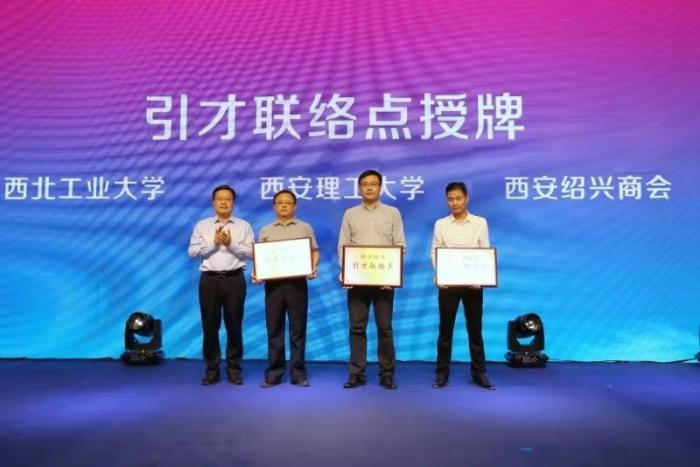2.校际、校地合作签协3.校企就业实习实践基地授牌